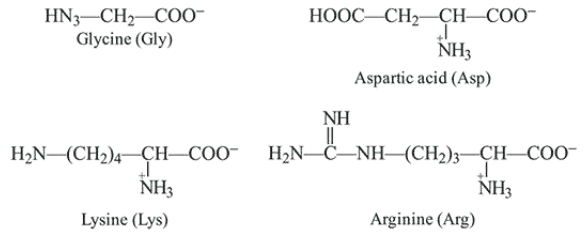# The increasing order of pKa of the following amino acids in aqueous solution is:

Question:

The increasing order of pKa of the following amino acids in aqueous solution is:

Gly Asp Lys Arg

1. Asp $<$ Gly $<$ Arg $<$ Lys

2. Gly $<$ Asp $<$ Arg $<$ Lys

3. Asp $<$ Gly $<$ Lys $<$ Arg

4. Arg $<$ Lys $<$ Gly $<$ Asp

Correct Option:

Solution:

Structure of the given $\alpha$-amino acids are:Here, aspartic acid is an acidic and glycine is a neutral amino acid while lysine and arginine are basic amino acids. Also, arginine is more basic due to the stronger basic functional groups.

$\therefore$ The order of $p K a$ value is directly proportional to the basic strength of amino acids, i.e. Arg $>$ Lys $>$Gly > Asp.